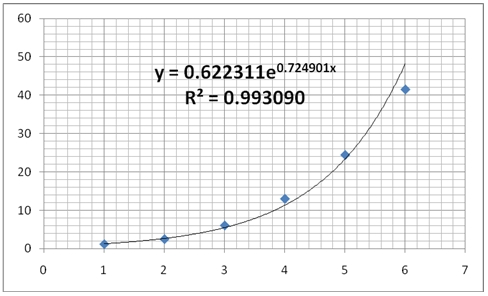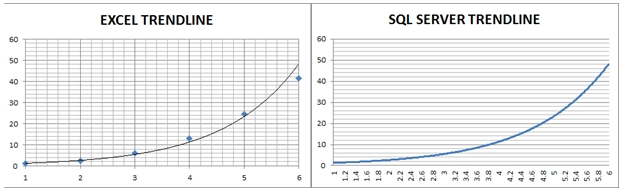## Calculating Exponential Trendline Values in SQL Server

May 14

Written by: Charles Flock
5/14/2011 1:22 PMIn this posting we demonstrate how to replicate the EXCEL Exponential Trendline in SQL Server using XLeratorDB.

 x y 1 1.1875 2 2.5 3 6.0625 4 13 5 24.4375 6 41.5

We then create a graph of the x- and y-values in EXCEL and add a trendline, using the Exponential option.

This produces the following chart.This chart contains the plotted points of our x- and y-values, and has calculated an exponential trendline. The equation for that trendline is:
y = 0.622311e0.724901x
Which means that each y-value is calculated using this equation. For example, at x = 1.5
y = 0.622311e0.724901(1.5)
y = 1.846027

In XLeratorDB we have several functions that enable you to do precisely these types of calculations on the database. By using the SLOPE and INTERECPT function we can calculate the coefficient and exponent for the trendline equation
Let’s put our x- and y-values into a temporary table (just to keep things simple), and calculate the coefficient and the exponent.
SET NOCOUNT ON

SELECT *
INTO #xy
FROM (VALUES
(1,1.1875),
(2,2.5),
(3,6.0625),
(4,13),
(5,24.4375),
(6,41.5)
) n(x,y)

SELECT ROUND(EXP(wct.INTERCEPT(LOG(y),x)), 6) as coefficient
,ROUND(wct.SLOPE(LOG(y),x), 6) as exponent
FROM #xy

This produces the following result.
coefficient               exponent
---------------------- ----------------------
0.622311               0.724901

These values equal the values produced by the EXCEL trendline.

We are now in a position to evaluate y at any value of x, using the coefficient and the exponent. Using the temporary table, we can simply evaluate y at x = 1.5.
SELECT ROUND(EXP(wct.INTERCEPT(LOG(y),x)) * EXP(wct.SLOPE(LOG(y),x) * CAST(1.5 as float)), 6) as y
FROM #xy
This produces the following result.
y
----------------------
1.846027

This is exactly the result we would expect based on the exponential formula.

We can evaluate y at any x, with some very simple SQL. Let’s say we wanted to evaluate y at a number of points.
SELECT xnew as x
,ROUND(EXP(wct.INTERCEPT(LOG(y),x)) * EXP(wct.SLOPE(LOG(y),x) * CAST(xnew as float)), 6) as y
FROM (VALUES
(0.5),(1),(1.5),(2),(2.5),(3),(3.5),(4),(4.5),(5),(5.5),(6),(6.5)
) m(xnew), #xy
GROUP BY xnew

This produces the following result.
x                      y
---------------------- ----------------------
0.5               0.894165
1.0               1.284777
1.5               1.846027
2.0               2.652457
2.5               3.811172
3.0               5.476067
3.5               7.868264
4.0              11.305483
4.5              16.244238
5.0              23.340467
5.5              33.536656
6.0              48.187007
6.5              69.237306

As you can see, we are able to evaluate the function for values of x which are greater than the maximum value of x supplied as input to the function. In our #xy table, x is in the range [1, 6], yet we are able to return results for new x-values greater than 6. The same thing is true for values less than 1.

While it is possible to generate trendline values where the supplied x is less than zero, it is not possible to evaluate an exponential trendline where there y-values input to the calculation that are less than or equal to zero, as the LOG function is undefined at those points. EXCEL will not allow you to generate a trendline for a range containing zero or negative y-values for this reason.

In this example, we will use the XLeratorDB number-generating function SERIESFLOAT to generate new x-values in the range [1, 6] in increments of .01. We will then take the results (which are not included here) and paste them into EXCEL and graph them. We can then compare the trendline values between EXCEL and SQL Server.
SELECT k.SeriesValue as xnew
,ROUND(EXP(wct.INTERCEPT(LOG(y),x)) * EXP(wct.SLOPE(LOG(y),x) * CAST(k.SeriesValue as float)), 6) as y
FROM #xy, wct.SeriesFloat(1,6,.01,NULL,NULL) k
GROUP BY k.SeriesValue
Here you can see a side-by-side comparison of the graphs.As you can see, the graphed results are identical.

In addition to the exponential coefficients, the EXCEL trendline function also gave us the option of displaying something called the R-squared value on the graph. We can calculate the R-squared value using the XLeratorDB RSQ function.
SELECT ROUND(wct.RSQ(LOG(y),x), 6) as [R-squared]
FROM #xy

This produces the following result.
R-squared
----------------------
0.99309

This matches the EXCEL calculation. You can think of the R-squared value as a measure of how well the trendline fits through the existing y-values.

The real value in these functions, however, results in the fact that they are aggregate functions, so they can handle hundreds of thousands, even millions of rows of data in a single unit of work. Where this becomes handy is when these millions of rows are going to be grouped in some meaningful way. For example, if you wanted to analyze the price movements for every symbol traded on the NYSE over the preceding 14 trading days, you could do that using an exponential trendline and you can use the RSQ function to give you some measure of the variability of the result.

Here’s a very simple example. We will create a 3-column table holding the symbol, date, and closing price for the last 14 trading days. We will use the SLOPE and INTERCEPT functions to predict the price by creating an exponential trendline and we will also calculate the R-squared value. We will only use 5 different symbols for this example.
SET NOCOUNT ON

/*Create the table*/
CREATE TABLE #c(
sym   char(3),
tdate datetime,
cprice float
)
/*Populate the table with data*/
INSERT INTO #c VALUES ('ABC','2011-04-01',6.64)
INSERT INTO #c VALUES ('DEF','2011-04-01',11.66)
INSERT INTO #c VALUES ('GHI','2011-04-01',10.66)
INSERT INTO #c VALUES ('JKL','2011-04-01',35.89)
INSERT INTO #c VALUES ('MNO','2011-04-01',113.79)
INSERT INTO #c VALUES ('ABC','2011-04-04',6.68)
INSERT INTO #c VALUES ('DEF','2011-04-04',11.77)
INSERT INTO #c VALUES ('GHI','2011-04-04',10.66)
INSERT INTO #c VALUES ('JKL','2011-04-04',35.89)
INSERT INTO #c VALUES ('MNO','2011-04-04',114.11)
INSERT INTO #c VALUES ('ABC','2011-04-05',6.74)
INSERT INTO #c VALUES ('DEF','2011-04-05',11.83)
INSERT INTO #c VALUES ('GHI','2011-04-05',10.74)
INSERT INTO #c VALUES ('JKL','2011-04-05',36.17)
INSERT INTO #c VALUES ('MNO','2011-04-05',115.08)
INSERT INTO #c VALUES ('ABC','2011-04-06',6.75)
INSERT INTO #c VALUES ('DEF','2011-04-06',11.9)
INSERT INTO #c VALUES ('GHI','2011-04-06',10.78)
INSERT INTO #c VALUES ('JKL','2011-04-06',36.39)
INSERT INTO #c VALUES ('MNO','2011-04-06',115.28)
INSERT INTO #c VALUES ('ABC','2011-04-07',6.78)
INSERT INTO #c VALUES ('DEF','2011-04-07',11.97)
INSERT INTO #c VALUES ('GHI','2011-04-07',10.87)
INSERT INTO #c VALUES ('JKL','2011-04-07',36.66)
INSERT INTO #c VALUES ('MNO','2011-04-07',116.29)
INSERT INTO #c VALUES ('ABC','2011-04-08',6.82)
INSERT INTO #c VALUES ('DEF','2011-04-08',12)
INSERT INTO #c VALUES ('GHI','2011-04-08',10.96)
INSERT INTO #c VALUES ('JKL','2011-04-08',36.89)
INSERT INTO #c VALUES ('MNO','2011-04-08',116.8)
INSERT INTO #c VALUES ('ABC','2011-04-11',6.88)
INSERT INTO #c VALUES ('DEF','2011-04-11',12.11)
INSERT INTO #c VALUES ('GHI','2011-04-11',10.99)
INSERT INTO #c VALUES ('JKL','2011-04-11',37.1)
INSERT INTO #c VALUES ('MNO','2011-04-11',117.53)
INSERT INTO #c VALUES ('ABC','2011-04-12',6.92)
INSERT INTO #c VALUES ('DEF','2011-04-12',12.21)
INSERT INTO #c VALUES ('GHI','2011-04-12',11.09)
INSERT INTO #c VALUES ('JKL','2011-04-12',37.31)
INSERT INTO #c VALUES ('MNO','2011-04-12',117.75)
INSERT INTO #c VALUES ('ABC','2011-04-13',6.98)
INSERT INTO #c VALUES ('DEF','2011-04-13',12.31)
INSERT INTO #c VALUES ('GHI','2011-04-13',11.19)
INSERT INTO #c VALUES ('JKL','2011-04-13',37.35)
INSERT INTO #c VALUES ('MNO','2011-04-13',118.33)
INSERT INTO #c VALUES ('ABC','2011-04-14',6.99)
INSERT INTO #c VALUES ('DEF','2011-04-14',12.32)
INSERT INTO #c VALUES ('GHI','2011-04-14',11.28)
INSERT INTO #c VALUES ('JKL','2011-04-14',37.61)
INSERT INTO #c VALUES ('MNO','2011-04-14',118.51)
INSERT INTO #c VALUES ('ABC','2011-04-15',7.04)
INSERT INTO #c VALUES ('DEF','2011-04-15',12.34)
INSERT INTO #c VALUES ('GHI','2011-04-15',11.33)
INSERT INTO #c VALUES ('JKL','2011-04-15',37.69)
INSERT INTO #c VALUES ('MNO','2011-04-15',118.94)
INSERT INTO #c VALUES ('ABC','2011-04-18',7.08)
INSERT INTO #c VALUES ('DEF','2011-04-18',12.43)
INSERT INTO #c VALUES ('GHI','2011-04-18',11.4)
INSERT INTO #c VALUES ('JKL','2011-04-18',38.04)
INSERT INTO #c VALUES ('MNO','2011-04-18',119.08)
INSERT INTO #c VALUES ('ABC','2011-04-19',7.14)
INSERT INTO #c VALUES ('DEF','2011-04-19',12.51)
INSERT INTO #c VALUES ('GHI','2011-04-19',11.4)
INSERT INTO #c VALUES ('JKL','2011-04-19',38.24)
INSERT INTO #c VALUES ('MNO','2011-04-19',120.23)
INSERT INTO #c VALUES ('ABC','2011-04-20',7.21)
INSERT INTO #c VALUES ('DEF','2011-04-20',12.53)
INSERT INTO #c VALUES ('GHI','2011-04-20',11.46)
INSERT INTO #c VALUES ('JKL','2011-04-20',38.52)
INSERT INTO #c VALUES ('MNO','2011-04-20',120.63)
/*Calculate the results*/
SELECT sym
,ROUND(EXP(wct.INTERCEPT(LOG(y),x)) * EXP(wct.SLOPE(LOG(y),x) * CAST(14 as float)), 6) as [Predicted Value]
,ROUND(wct.RSQ(LOG(y),x), 6) as [R-squared]
FROM (
SELECT sym
,ROW_NUMBER() OVER (PARTITION BY sym ORDER BY tdate) - 1 as x
,cprice as y
FROM #c
) n
GROUP by n.sym
/*Clean up*/
DROP TABLE #c

This produces the following result.
sym         Predicted Value              R-squared
---- ---------------------- ----------------------
ABC                7.223715               0.992419
DEF               12.649476               0.986554
GHI               11.580442               0.986482
JKL                38.66916               0.991772
MNO              121.213892               0.982004

You will notice that we used the ROW_NUMBER() function instead of passing dates to the aggregate functions. In general, because of the limits of floating point math, you will get the best results by reducing datetime values to values representing the elapsed time from some starting point (this also happens to be true with EXCEL trendline). In fact, in this example, we just used trading dates, so we were unconcerned about the weekends and we counted the number of days from a Friday to a Monday as 1 (and not 3).

Calculating exponential trendline values can be an important tool, either filling in the gaps between known x-values, or for predicting an outcome for some new x-value. And XLeratorDB makes it simple and efficient to do these calculations right on the database, where your data is.

Tags:
Categories:
Location: BlogsThe WestClinTech Blog

## Search Blogs

 KeywordsPhrase

## Blog Archives

Archive
 < May 2023 >
SunMonTueWedThuFriSat
30123456
78910111213
14151617181920
21222324252627
28293031123
45678910
Monthly
Go

### SupportCopyright 2008-2023 Westclintech LLC         Privacy Policy        Terms of Service